Sequential satisficing

In our last post, we ran simulations on our 1,000 randomly generated return scenarios to compare the average and risk-adjusted return for satisfactory, naive, and mean-variance optimized (MVO) maximum return and maximum Sharpe ratio portfolios.1 We found that you can shoot for high returns or high risk-adjusted returns, but rarely both. Assuming no major change in the underlying average returns and risk, choosing the efficient high return or high risk-adjusted return portfolio generally leads to similar performance a majority of the time in out-of-sample simulations. What was interesting about the results was that even though we weren’t arguing that one should favor satisficing over optimizing, we found that the satisfactory portfolio generally performed well.

One area we didn’t test was the effect of sequence on mean-variance optimization. Recall we simulated 1,000 different sixty month (five year) return profiles for four potential assets—stocks, bonds, commodities (gold) and real estate. The weights we used to calculate the different portfolio returns and risk were based on data from 1987-1991 and were kept constant for all the simulations. What if the weighting system could “learn” from previous scenarios or adjust to recent information?

In this post, we’ll look at the impact of incorporating sequential information on portfolio results and compare that to the non-sequential results. To orient folks, we’ll show the initial portfolio weight simulation graph with the different portfolios and efficient frontier based on the historical data. The satisfactory, naive, MVO-derived maximum Sharpe ratio, and MVO-derived maximum return portfolios are the colored blue, black, red, and purple points, respectively.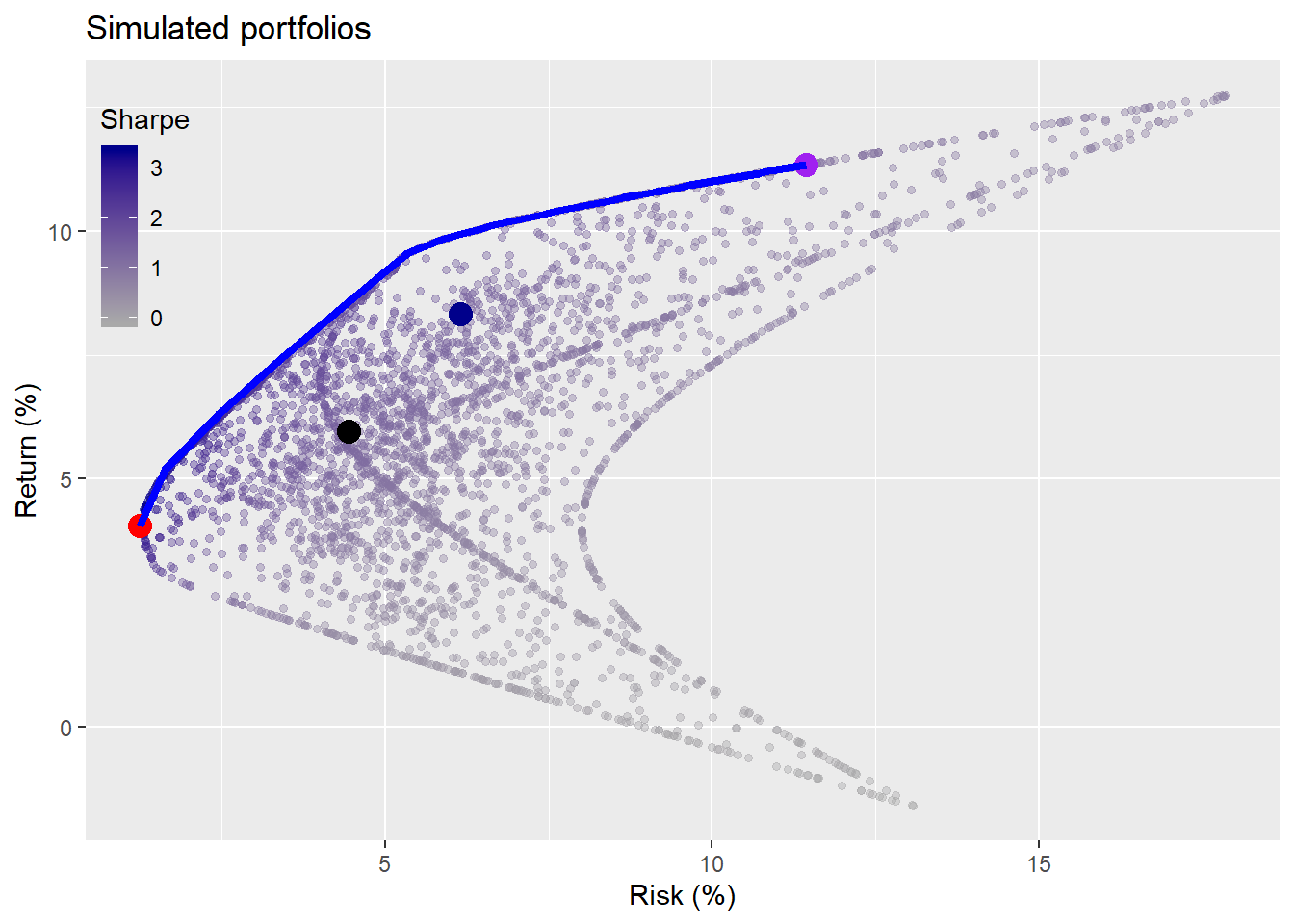Now we’ll take the weighting schemes and apply them to the return simulations. However, we’ll run through the returns sequentially and calculate the returns and risk-adjusted returns for the weighting scheme using the prior simulation as the basis for the allocation on the next simulation. For example, we’ll calculate the efficient frontier and run the portfolio weight algorithm (see Weighting on a friend for more details) on simulation #75. From those calculations, we’ll assign the weights for the satisfactory, maximum Sharpe ratio, and maximum efficient return portfolios to compute the returns and risk-adjusted returns on simulation #76. For the record, simulation #1 uses the same weights as those that produced the dots in the graph above.

Running the simulations, here’s the average return by weighting algorithm.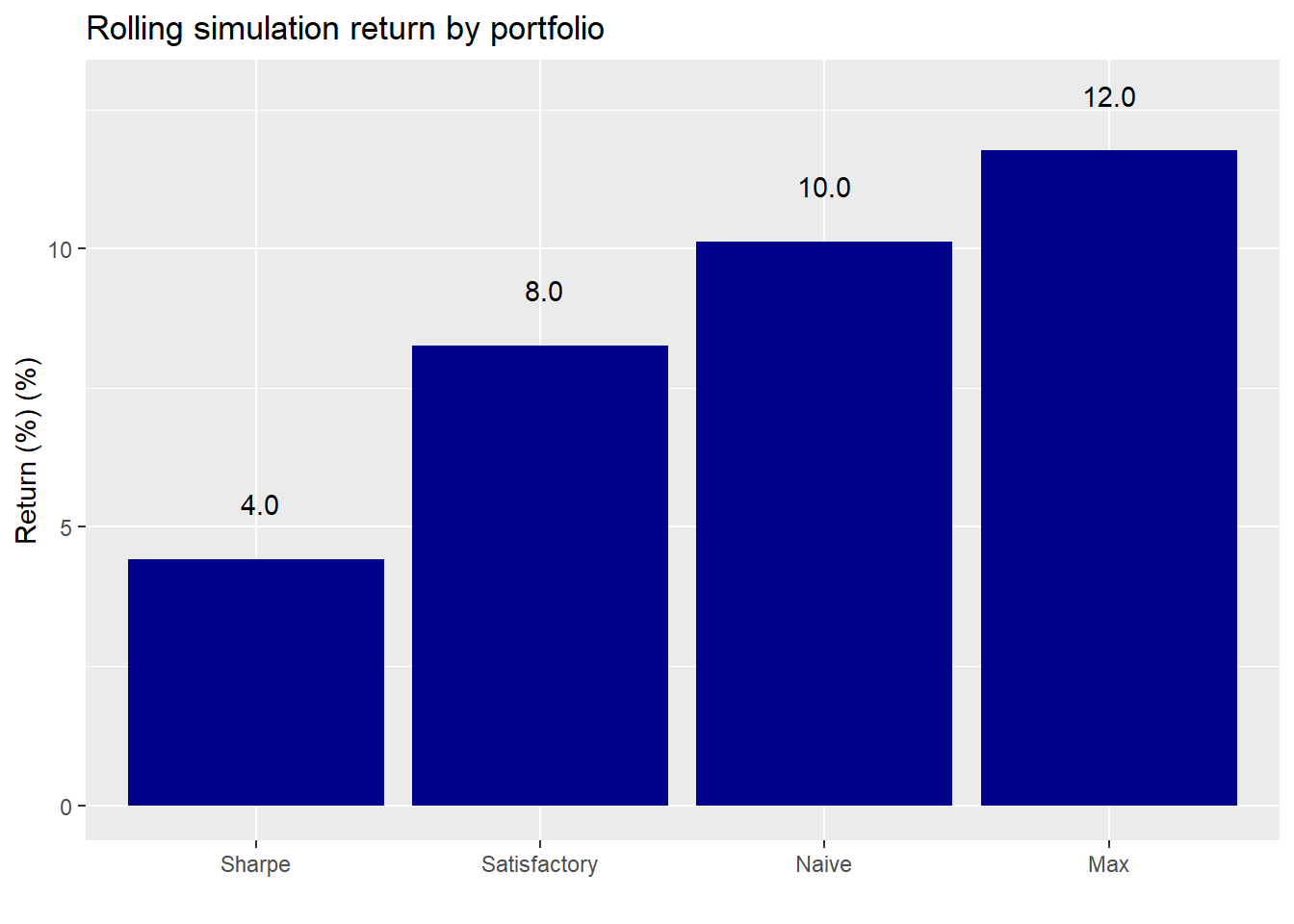And here’s the original.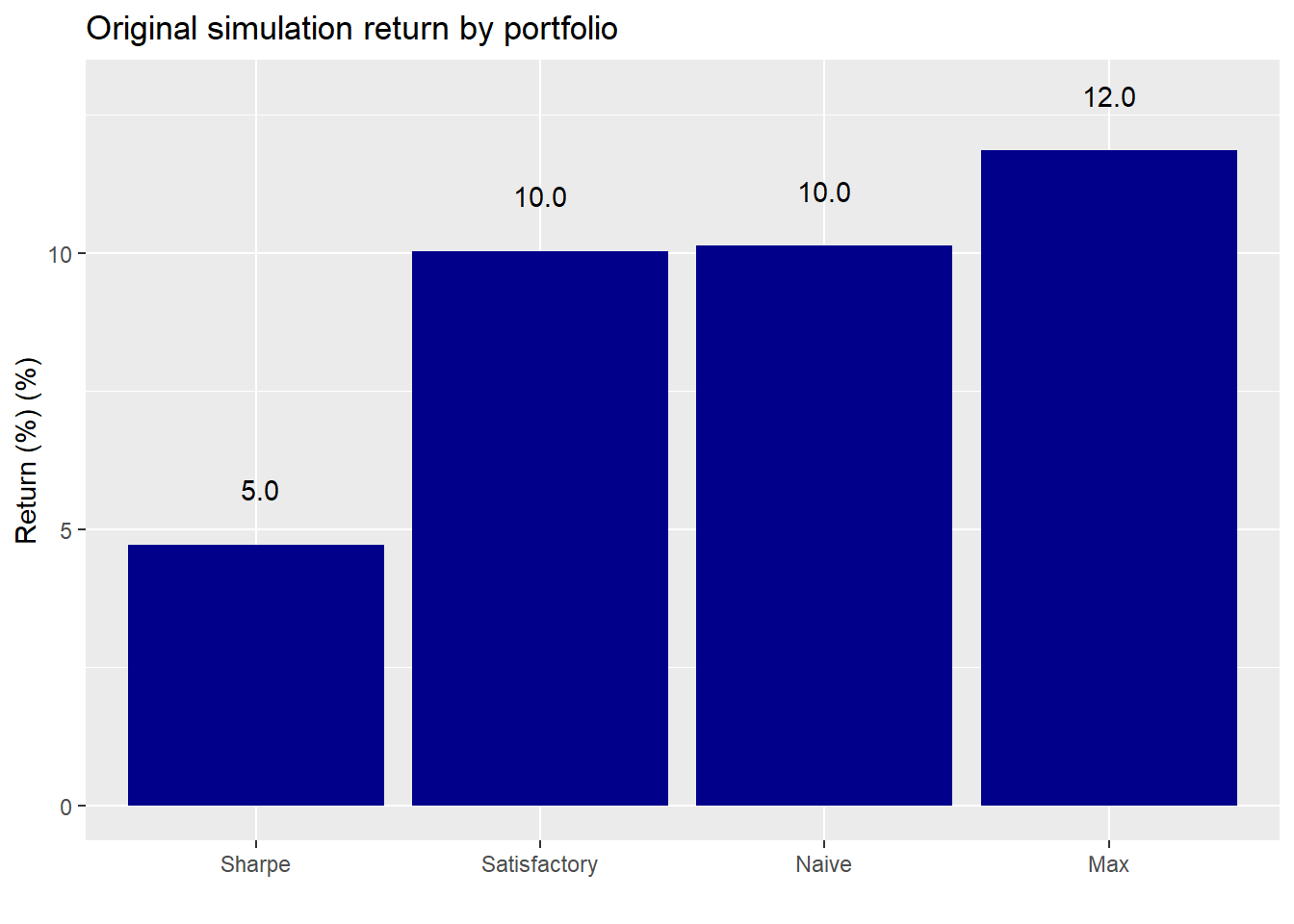For the naive and maximum return portfolios, the average return isn’t much different on the sequential calculations than on original constant weight computations. The sequential Sharpe allocations are about 1% point lower than the stable ones. However, the sequential satisfactory allocations are almost 2% points lower than the constant allocation. In about 5% of the cases, the return simulation was not capable of achieving a satisfactory portfolio with the original constraints of not less than 7% and not more than 10% annualized return and risk. In such a case, we reverted to the original weighting. This adjustment did not affect the average return even when we excluded the “unsatisfactory” portfolios.

We assume that the performance drag is due to the specificity of the constraints amid random outcomes. The other portfolios (except for the naive one) optimize for the previous scenario and apply those optimal weights on the next portfolio in the sequence. The satisfactory portfolio chooses average weights to achieve a particular constraint and then applies those weights in sequence. Due to the randomness of the return scenarios, it seems possible that a weighting scheme that works for one scenario would produce poor results on another scenario. Of course, the satisfactory portfolios still achieved their return constraint on average.

This doesn’t exactly explain why the MVO portfolios didn’t suffer a performance drag. Our intuition is that the MVO portfolios are border cases. Hence, there probably won’t be too much dispersion in the results on average since the return simulations draw from the same return, risk, and error terms throughout. However, the satisfactory portfolio lies with the main portion of the territory, so the range of outcomes could be much larger. Clearly, this requires further investigation, but we’ll have to shelve that for now.

In the next set of graphs, we check out how each portfolio performs relative to the others. First the sequential simulation.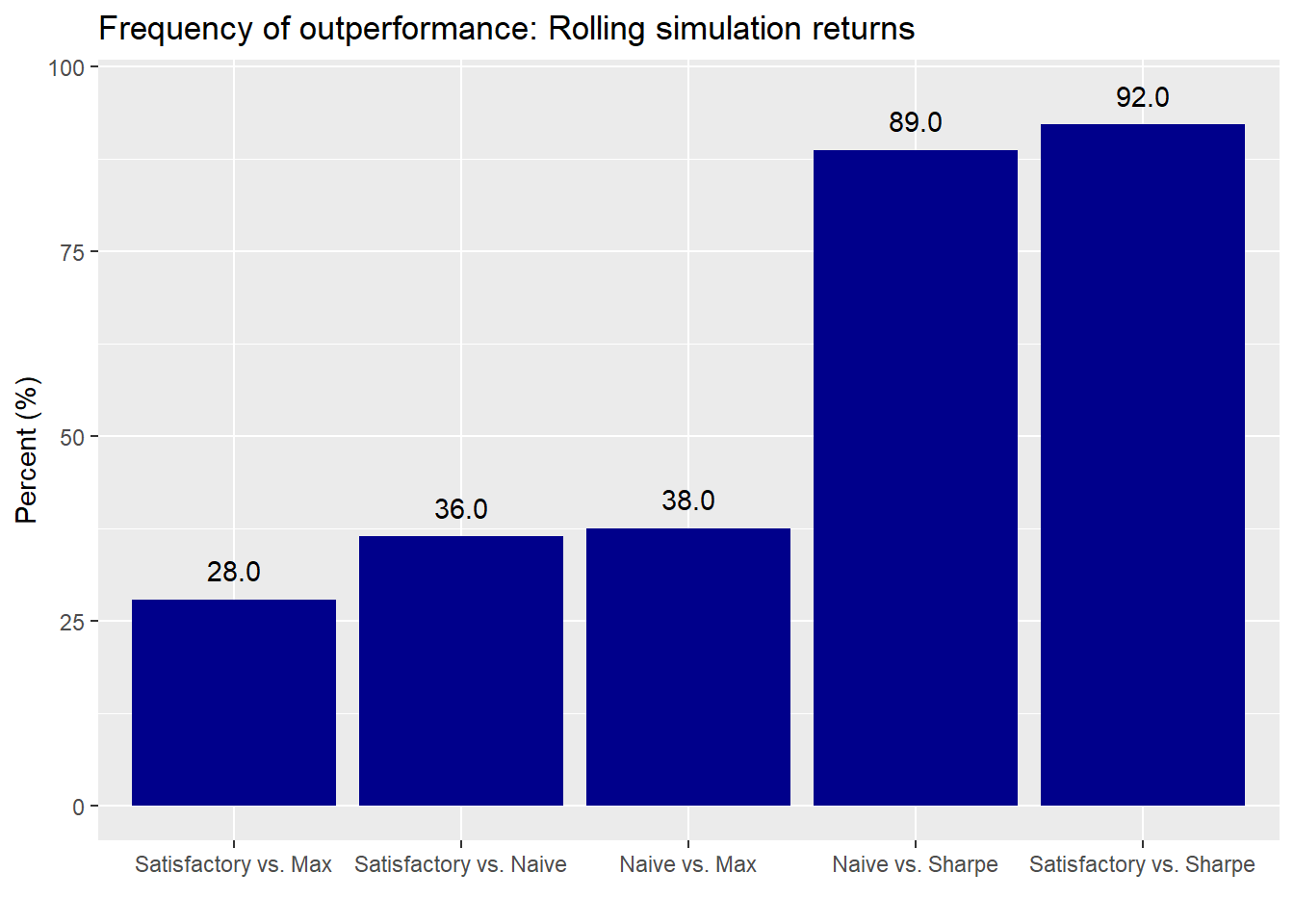And the original: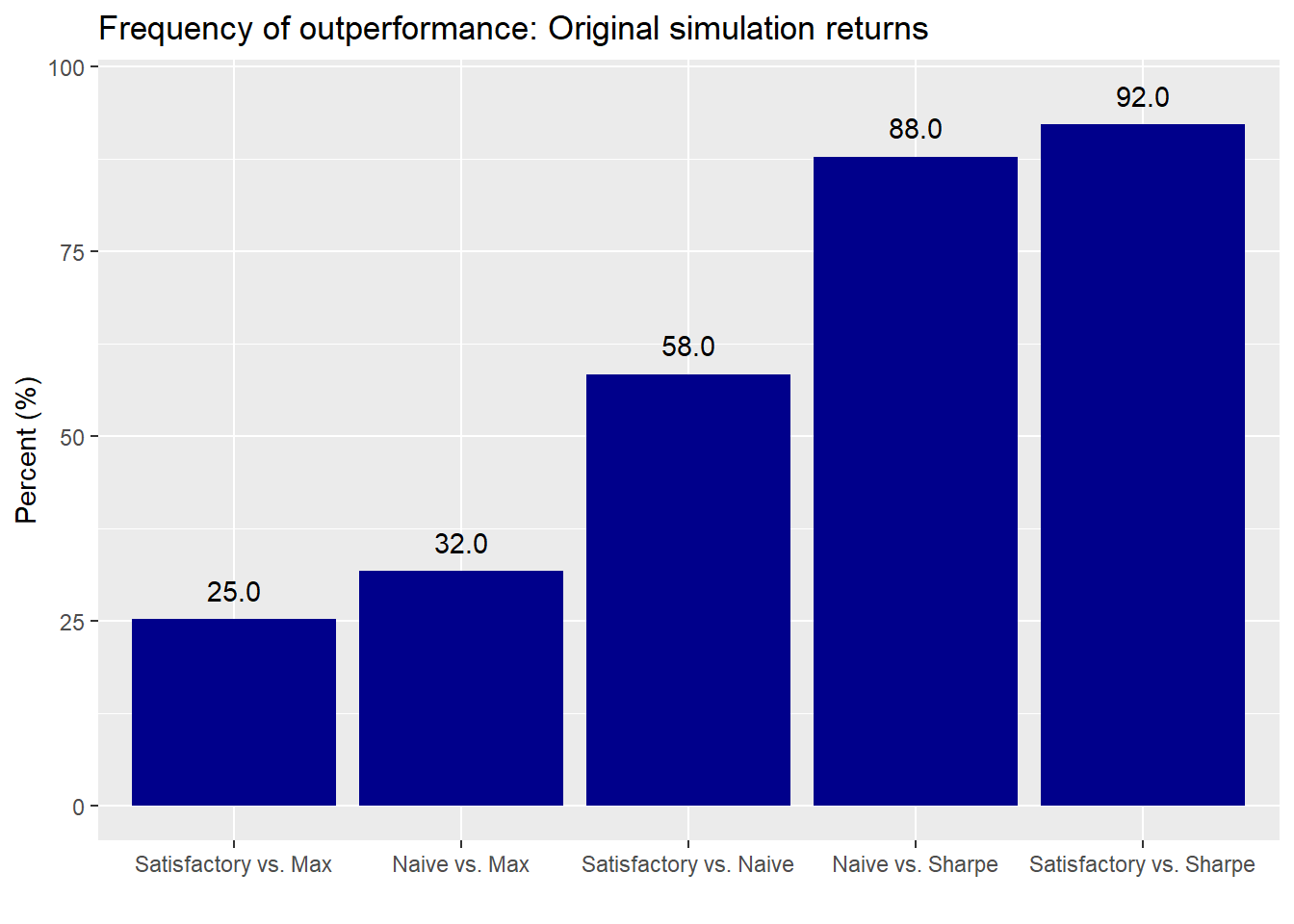There are some modest changes in the satisfactory and naive vs. MVO-derived portfolios. But the biggest change occurs in the satisfactory vs. naive portfolio—almost a 22 percentage point decline! This appears to support our conjecture above that the order of the sequence could be affecting the satisfactory portfolio’s performance. Nonetheless, sequencing does not appear to alter the performance of the naive and satisfactory portfolios relative to the MVO-derived ones in a material way. Let’s randomize the sequence to say if results change.

For the random draw case, we randomly draw one return simulation on which to calculate our portfolio weights and then apply those weights on another randomly drawn portfolio. The draws are without replacement to ensure we don’t use the same simulation both to calculate weights and returns as well as to ensure that we use all simulations.2

The average return graph isn’t much different for the random sampling than it is for the sequential so we’ll save some space and not print it. Here is the relative performance graph.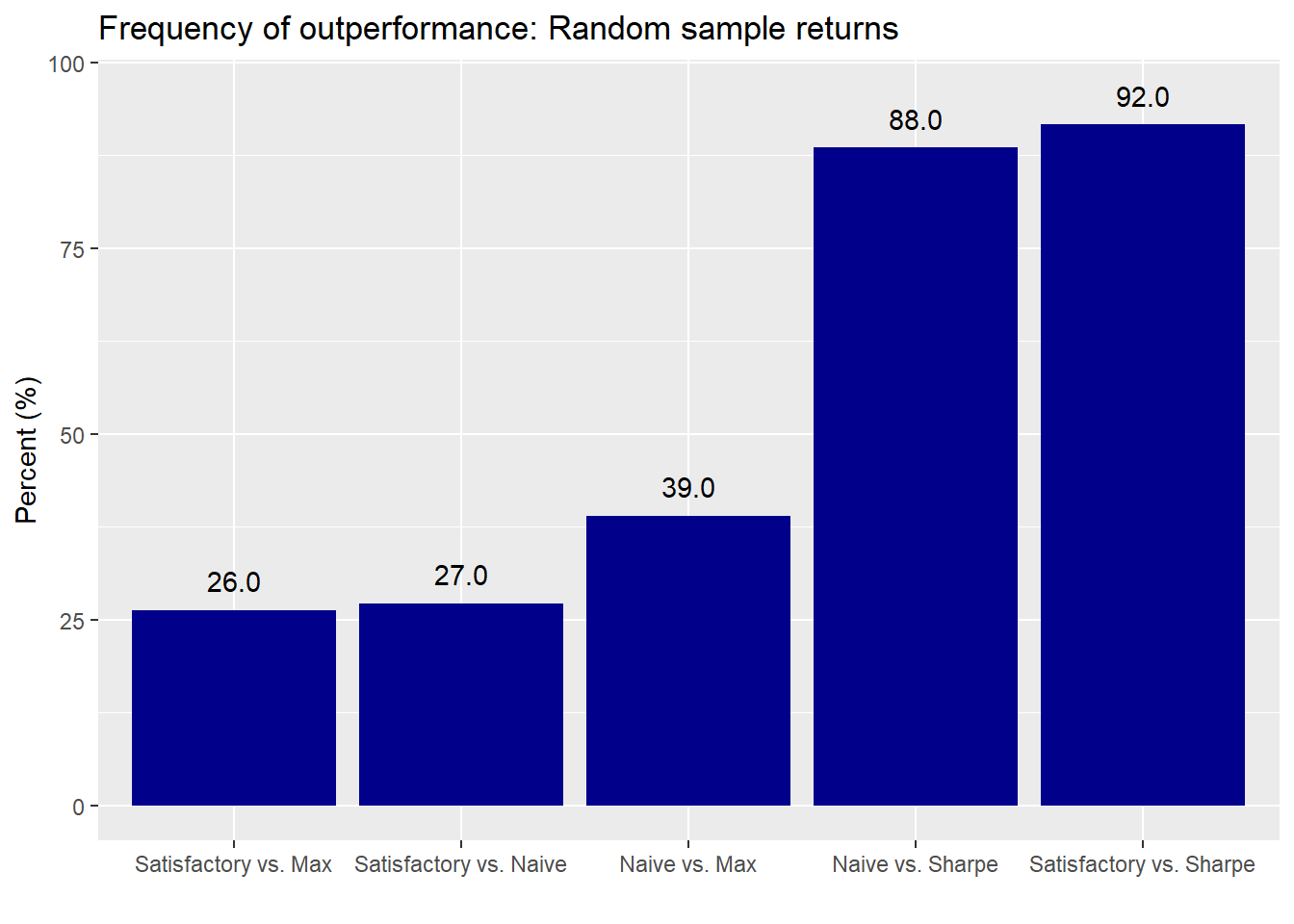And here is the original relative performance for ease of comparison.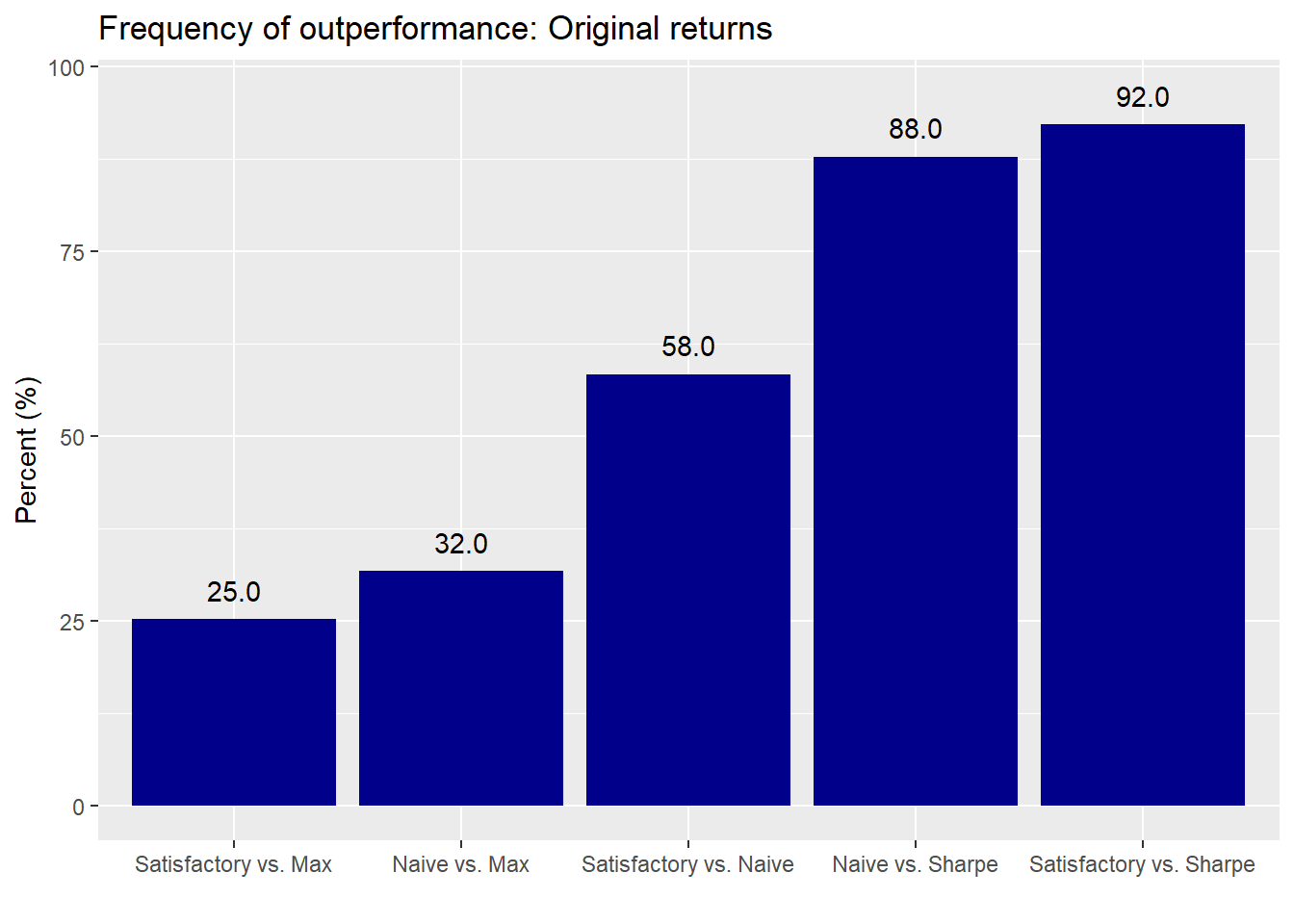With random ordering we see that the naive and satisfactory portfolios relative to the maximum return experience a modest performance improvement. Relative to the maximum Sharpe portfolio, the performance is relatively unchanged. The satisfactory portfolio again suffers a drag relative to the naive, but somewhat less severe that in the sequential case.

What are the takeaways? In both sequential and random sampling, the performance of the naive and satisfactory compared to the MVO portfolios was relatively stable. That supports our prior observation that you can shoot for high returns or high risk-adjusted returns, but usually not both. It’s also noteworthy that the maximum Sharpe ratio consistently underperforms the naive and satisfactory portfolios in all of the simulations. The team at Alpha Architect noted similar results in a much broader research project. Here, part of the study found that the maximum Sharpe portfolio suffered the worst performance relative to many others including an equal-weighted (i.e., naive) portfolio.

One issue with our random sampling test is that it was only one random sequence. To be truly robust, we’d want to run the sampling simulation more than once. But we’ll have to save that for when we rent time on a cloud GPU because we don’t think our flintstone-aged laptop will take kindly to running 1,000 simulations of 1,000 random sequences of 1,000 simulated portfolios with 1,000 optimizations and 3,000 random portfolio weightings!

Perceptive readers might quibble with how we constructed our “learning” simulations. Indeed, in both the sequential and random sample cases we calculated our allocation weights only using the prior simulation in the queue (e.g. weights derived from return simulation #595 are deployed on simulation #596 (or, for example, #793 in the random simulation)). But that “learning” could be considered relatively short term. A more realistic scenario would be to allow for cumulative learning by trying to incorporate all or a majority of past returns in the sequence. And we could get even more complicated by throwing in some weighting scheme to emphasize nearer term results. Finally, we could calculate cumulative returns over one scenario or multiple scenarios. We suspect relative performance would be similar, but you never know!

Until we experiment with these other ideas, the R and Python code are below. While we generally don’t discuss the code in detail, we have been getting more questions and constructive feedback on it of late. Thank you for that! One thing to note is the R code to produce the simulations runs much quicker than the Python code. Part of that is likely due to how we coded in the efficient frontier and maximum Sharpe and return portfolios in the different languages. But the differences are close to an order of magnitude. If anyone notices anything we could do to improve performance, please drop us an email at nbw dot osm at gmail dot com. Thanks!

R code

# Built using R 3.6.2

suppressPackageStartupMessages({
library(tidyquant)
library(tidyverse)
})

# Seem prior posts for how we built these data frames
sym_names <- c("stock", "bond", "gold", "realt", "rfr")

### Call functions
# See prior posts for how we built these functions
source("Portfolio_simulation_functions.R")
source("Efficient_frontier.R")

## Function for calculating satisfactory weighting
port_sim_wts <- function(df, sims, cols, return_min, risk_max){

if(ncol(df) != cols){
print("Columns don't match")
break
}

# Create weight matrix
wts <- matrix(nrow = (cols-1)*sims, ncol = cols)
count <- 1

for(i in 1:(cols-1)){
for(j in 1:sims){
a <- runif((cols-i+1),0,1)
b <- a/sum(a)
c <- sample(c(b,rep(0,i-1)))
wts[count,] <- c
count <- count+1
}

}

# Find returns
mean_ret <- colMeans(df)

# Calculate covariance matrix
cov_mat <- cov(df)

# Calculate random portfolios
port <- matrix(nrow = (cols-1)*sims, ncol = 2)
for(i in 1:nrow(port)){
port[i,1] <- as.numeric(sum(wts[i,] * mean_ret))
port[i,2] <- as.numeric(sqrt(t(wts[i,]) %*% cov_mat %*% wts[i,]))

}

port <- as.data.frame(port) %>%
colnames<-(c("returns", "risk"))

port_select  <-  cbind(port, wts)

port_wts <- port_select %>%
mutate(returns = returns*12,
risk = risk*sqrt(12)) %>%
filter(returns >= return_min,
risk <= risk_max) %>%
summarise_at(vars(3:6), mean)

port_wts

}

## Portfolio func
port_func <- function(df,wts){
mean_ret = colMeans(df)
returns = sum(mean_ret*wts)
risk = sqrt(t(wts) %*% cov(df) %*% wts)
c(returns, risk)
}

## Portfolio graph
pf_graf <- function(df, nudge, multiplier, rnd, y_lab, text){
df %>%
gather(key, value)  %>%
ggplot(aes(reorder(key, value), value*multiplier*100)) +
geom_bar(stat='identity',
fill = 'darkblue') +
geom_text(aes(label = format(round(value*multiplier,rnd)*100,nsmall = 1)), nudge_y = nudge)+
labs(x = "",
y = paste(y_lab, "(%)", sep = " "),
title = paste(text, "by portfolio", sep = " "))
}

## Create outperformance graph
perf_graf <- function(df, rnd, nudge, text){
df %>%
rename("Satisfactory vs. Naive" = ovs,
"Satisfactory vs. Max" = ovr,
"Naive vs. Max" = rve,
"Satisfactory vs. Sharpe" = ove,
"Naive vs. Sharpe" = sve) %>%
gather(key, value)  %>%
ggplot(aes(reorder(key, value), value*100)) +
geom_bar(stat='identity',
fill = 'darkblue') +
geom_text(aes(label = format(round(value,rnd)*100, nsmall = 1)), nudge_y = nudge)+
labs(x = "",
y = "Percent (%)",
title = paste("Frequency of outperformance:", text, sep = " "))

}

### Create weight schemes
satis_wts <- c(0.32, 0.4, 0.08, 0.2) # Calculated in previous post using port_select_func
simple_wts <- rep(0.25, 4)
eff_port <- eff_frontier_long(df[2:61,2:5], risk_increment = 0.01)
eff_sharp_wts <- eff_port[which.max(eff_port$sharpe),1:4] %>% as.numeric() eff_max_wts <- eff_port[which.max(eff_port$exp_ret), 1:4] %>% as.numeric()

## Run port sim on 1987-1991 data
port_sim_1 <- port_sim_lv(df[2:61,2:5],1000,4)

# Run function on three weighting schemes and one simulation
weight_list <- list(satis = satis_wts,
naive = simple_wts,
sharp = eff_sharp_wts,
max = eff_max_wts)

wts_df <- data.frame(wts = c("satis", "naive", "sharp", "max"), returns = 1:4, risk = 5:8,
stringsAsFactors = FALSE)
for(i in 1:4){
wts_df[i, 2:3] <- port_func(df[2:61,2:5], weight_list[[i]])

}

wts_df$sharpe = wts_df$returns/wts_df$risk # Graph portfolio simulation with three portfolios port_sim_1$graph +
geom_point(data = wts_df,
aes(x = risk*sqrt(12)*100, y = returns*1200),
color = c("darkblue", "black", "red", "purple"),
size = 4) +
geom_line(data = eff_port,
aes(stdev*sqrt(12)*100, exp_ret*1200),
color = 'blue',
size = 1.5) +
theme(legend.position = c(0.05,0.8), legend.key.size = unit(.5, "cm"),
legend.background = element_rect(fill = NA))

## Calculate performance with rolling frontier on max sharpe
set.seed(123)

eff_sharp_roll <- list()
eff_max_roll <- list()
satis_roll <- list()
for(i in 1:1000){

if(i == 1){
sharp_wts <- eff_sharp_wts
max_wts <- eff_max_wts
sat_wts <- satis_wts

}else {
eff_calc <- eff_frontier_long(sim1[[i-1]]$df, risk_increment = 0.01) sharp_wts <- eff_calc[which.max(eff_calc$sharpe), 1:4]
max_wts <- eff_calc[which.max(eff_calc$exp_ret), 1:4] sat_wts <- port_sim_wts(sim1[[i-1]]$df, 1000, 4, 0.07, 0.1)

}

eff_sharp_roll[[i]] <- port_func(sim1[[i]]$df, as.numeric(sharp_wts)) eff_max_roll[[i]] <- port_func(sim1[[i]]$df, as.numeric(max_wts))
satis_roll[[i]] <- port_func(sim1[[i]]$df, as.numeric(sat_wts)) } list_to_df <- function(list_ob){ x <- do.call('rbind', list_ob) %>% as.data.frame() %>% colnames<-(c("returns", "risk")) %>% mutate(sharpe = returns/risk) x } roll_lists <- c("eff_sharp_roll", "eff_max_roll", "satis_roll") for(obj in roll_lists){ x <- list_to_df(get(obj)) assign(obj, x) } # Return roll_mean_pf <- data.frame(Satisfactory = mean(satis_roll[,1], na.rm = TRUE), Naive = mean(simple_df[,1]), Sharpe = mean(eff_sharp_roll[,1]), Max = mean(eff_max_roll[,1])) # Graph mean returns pf_graf(roll_mean_pf, 1, 12, 2,"Return (%)", "Rolling simulation return") pf_graf(mean_pf, 1, 12, 2,"Return (%)", "Original simulation return") # Create relative performance df roll_ret_pf <- data.frame(ovs = mean(satis_df[,1] > simple_df[,1]), ovr = mean(satis_df[,1] > eff_max_roll[,1]), rve = mean(simple_df[,1] > eff_max_roll[,1]), ove = mean(satis_df[,1] > eff_sharp_roll[,1]), sve = mean(simple_df[,1] > eff_sharp_roll[,1])) # Graph outperformance perf_graf(roll_ret_pf, 2, 4, "Rolling simulation returns") perf_graf(ret_pf, 2, 4, "Original simulation returns") ## Sampling portfolios set.seed(123) eff_sharp_samp <- list() eff_max_samp <- list() satis_samp <- list() for(i in 1:1000){ if(i == 1){ sharp_wts <- eff_sharp_wts max_wts <- eff_max_wts sat_wts <- satis_wts }else { samp_1 <- sample(1000, 1) # Sample a return simulation for weight scheme eff_calc <- eff_frontier_long(sim1[[samp_1]]$df, risk_increment = 0.01)
sharp_wts <- eff_calc[which.max(eff_calc$sharpe), 1:4] max_wts <- eff_calc[which.max(eff_calc$exp_ret), 1:4]
sat_wts <- port_sim_wts(sim1[[samp_1]]$df, 1000, 4, 0.07, 0.1) } samp_2 <- sample(1000, 1) # sample a return simulation to analyze performance eff_sharp_samp[[i]] <- port_func(sim1[[samp_2]]$df, as.numeric(sharp_wts))
eff_max_samp[[i]] <- port_func(sim1[[samp_2]]$df, as.numeric(max_wts)) satis_samp[[i]] <- port_func(sim1[[samp_2]]$df, as.numeric(sat_wts))
}

samp_lists <- c("eff_sharp_samp", "eff_max_samp", "satis_samp")

for(obj in samp_lists){
x <- list_to_df(get(obj))
assign(obj, x)
}

# Return
samp_mean_pf <- data.frame(Satisfactory = mean(satis_samp[,1], na.rm=TRUE),
Naive = mean(simple_df[,1]),
Sharpe = mean(eff_sharp_samp[,1]),
Max = mean(eff_max_samp[,1]))

# Graph mean returns: NOT SHOWN
pf_graf(samp_mean_pf, 1, 12, 2,"Return (%)", "Random sampling return")

# pf_graf(mean_pf, 1, 12, 2,"Return (%)", "Original return")

# Create relative performance df
samp_ret_pf <- data.frame(ovs = mean(satis_df[,1] > simple_df[,1]),
ovr = mean(satis_df[,1] > eff_max_samp[,1]),
rve = mean(simple_df[,1] > eff_max_samp[,1]),
ove = mean(satis_df[,1] > eff_sharp_samp[,1]),
sve = mean(simple_df[,1] > eff_sharp_samp[,1]))

# Graph outperformance
perf_graf(samp_ret_pf, 2, 4, "Random sample returns")

perf_graf(ret_pf, 2, 4, "Original returns")

Python code

# Built using Python 3.7.4

import pandas as pd
import numpy as np
import matplotlib.pyplot as plt
%matplotlib inline

plt.style.use('ggplot')

# Seem prior posts for how we built these data frames
port_names = ['Original','Naive', 'Sharpe', 'Max']

# Portfolio simulation functions
# NOte the Port_sim class is slightly different than previous posts,
# so we're reproducing it here.
# We were getting a lot of "numpy.float64 is not callable" errors
# due to overlapping names on variables and functions, so we needed
# to fix the code. If it still throws error, let us know if it does.

## Simulation function
class Port_sim:
def calc_sim(df, sims, cols):
wts = np.zeros((sims, cols))

for i in range(sims):
a = np.random.uniform(0,1,cols)
b = a/np.sum(a)
wts[i,] = b

mean_ret = df.mean()
port_cov = df.cov()

port = np.zeros((sims, 2))
for i in range(sims):
port[i,0] =  np.sum(wts[i,]*mean_ret)
port[i,1] = np.sqrt(np.dot(np.dot(wts[i,].T,port_cov), wts[i,]))

sharpe = port[:,0]/port[:,1]*np.sqrt(12)

return port, wts, sharpe

def calc_sim_lv(df, sims, cols):
wts = np.zeros(((cols-1)*sims, cols))
count=0

for i in range(1,cols):
for j in range(sims):
a = np.random.uniform(0,1,(cols-i+1))
b = a/np.sum(a)
c = np.random.choice(np.concatenate((b, np.zeros(i))),cols, replace=False)
wts[count,] = c
count+=1

mean_ret = df.mean()
port_cov = df.cov()

port = np.zeros(((cols-1)*sims, 2))
for i in range(sims):
port[i,0] =  np.sum(wts[i,]*mean_ret)
port[i,1] = np.sqrt(np.dot(np.dot(wts[i,].T,port_cov), wts[i,]))

sharpe = port[:,0]/port[:,1]*np.sqrt(12)

return port, wts, sharpe

def graph_sim(port, sharpe):
plt.figure(figsize=(14,6))
plt.scatter(port[:,1]*np.sqrt(12)*100, port[:,0]*1200, marker='.', c=sharpe, cmap='Blues')
plt.colorbar(label='Sharpe ratio', orientation = 'vertical', shrink = 0.25)
plt.title('Simulated portfolios', fontsize=20)
plt.xlabel('Risk (%)')
plt.ylabel('Return (%)')
plt.show()

# Constraint function
def port_select_func(port, wts, return_min, risk_max):
port_select = pd.DataFrame(np.concatenate((port, wts), axis=1))
port_select.columns = ['returns', 'risk', 1, 2, 3, 4]

port_wts = port_select[(port_select['returns']*12 >= return_min) & (port_select['risk']*np.sqrt(12) <= risk_max)]
port_wts = port_wts.iloc[:,2:6]
port_wts = port_wts.mean(axis=0)
return port_wts

def port_select_graph(port_wts):
plt.figure(figsize=(12,6))
key_names = {1:"Stocks", 2:"Bonds", 3:"Gold", 4:"Real estate"}
lab_names = []
graf_wts = port_wts.sort_values()*100

for i in range(len(graf_wts)):
name = key_names[graf_wts.index[i]]
lab_names.append(name)

plt.bar(lab_names, graf_wts, color='blue')
plt.ylabel("Weight (%)")
plt.title("Average weights for risk-return constraint", fontsize=15)

for i in range(len(graf_wts)):
plt.annotate(str(round(graf_wts.values[i])), xy=(lab_names[i], graf_wts.values[i]+0.5))

plt.show()

# We should have wrapped the three different efficient frontier functions
# into one class or function but ran out of time. This is probably what slows
# down the simulations below.

# Create efficient frontier function
from scipy.optimize import minimize

def eff_frontier(df_returns, min_ret, max_ret):

n = len(df_returns.columns)

def get_data(weights):
weights = np.array(weights)
returns = np.sum(df_returns.mean() * weights)
risk = np.sqrt(np.dot(weights.T, np.dot(df_returns.cov(), weights)))
sharpe = returns/risk
return np.array([returns,risk,sharpe])

# Contraints
def check_sum(weights):
return np.sum(weights) - 1

# Rante of returns
mus = np.linspace(min_ret,max_ret,21)

# Function to minimize
def minimize_volatility(weights):
return  get_data(weights)

# Inputs
init_guess = np.repeat(1/n,n)
bounds = ((0.0,1.0),) * n

eff_risk = []
port_weights = []

for mu in mus:
# function for return
cons = ({'type':'eq','fun': check_sum},
{'type':'eq','fun': lambda w: get_data(w) - mu})

result = minimize(minimize_volatility,init_guess,method='SLSQP',bounds=bounds,constraints=cons)

eff_risk.append(result['fun'])
port_weights.append(result.x)

eff_risk = np.array(eff_risk)

return mus, eff_risk, port_weights

# Create max sharpe function
from scipy.optimize import minimize

def max_sharpe(df_returns):

n = len(df_returns.columns)

def get_data(weights):
weights = np.array(weights)
returns = np.sum(df_returns.mean() * weights)
risk = np.sqrt(np.dot(weights.T, np.dot(df_returns.cov(), weights)))
sharpe = returns/risk
return np.array([returns,risk,sharpe])

# Function to minimize
def neg_sharpe(weights):
return  -get_data(weights)

# Inputs
init_guess = np.repeat(1/n,n)
bounds = ((0.0,1.0),) * n

# function for return
constraint = {'type':'eq','fun': lambda x: np.sum(x) - 1}

result = minimize(neg_sharpe,
init_guess,
method='SLSQP',
bounds=bounds,
constraints=constraint)

return -result['fun'], result['x']

# Create efficient frontier function
from scipy.optimize import minimize

def max_ret(df_returns):

n = len(df_returns.columns)

def get_data(weights):
weights = np.array(weights)
returns = np.sum(df_returns.mean() * weights)
risk = np.sqrt(np.dot(weights.T, np.dot(df_returns.cov(), weights)))
sharpe = returns/risk
return np.array([returns,risk,sharpe])

# Function to minimize
def port_ret(weights):
return  -get_data(weights)

# Inputs
init_guess = np.repeat(1/n,n)
bounds = ((0.0,1.0),) * n

# function for return
constraint = {'type':'eq','fun': lambda x: np.sum(x) - 1}

result = minimize(port_ret,
init_guess,
method='SLSQP',
bounds=bounds,
constraints=constraint)

return -result['fun'], result['x']

## Portfolio return
def port_func(df, wts):
mean_ret = df.mean()
returns = np.sum(mean_ret * wts)
risk = np.sqrt(np.dot(wts, np.dot(df.cov(), wts)))
return returns, risk

## Return and relative performance graph
def pf_graf(names, values, rnd, nudge, ylabs, graf_title):
df = pd.DataFrame(zip(names, values), columns = ['key', 'value'])
sorted = df.sort_values(by = 'value')
plt.figure(figsize = (12,6))
plt.bar('key', 'value', data = sorted, color='darkblue')

for i in range(len(names)):
plt.annotate(str(round(sorted['value'][i], rnd)), xy = (sorted['key'][i], sorted['value'][i]+nudge))

plt.ylabel(ylabs)
plt.title('{} performance by portfolio'.format(graf_title))
plt.show()

## Create portfolio
np.random.seed(123)
port_sim_1, wts_1, sharpe_1 = Port_sim.calc_sim(df.iloc[1:61,0:4],1000,4)

# Create returns and min/max ranges
df_returns = df.iloc[1:61, 0:4]
min_ret = min(port_sim_1[:,0])
max_ret = max(port_sim_1[:,0])

# Find efficient portfolio
eff_ret, eff_risk, eff_weights = eff_frontier(df_returns, min_ret, max_ret)
eff_sharpe = eff_ret/eff_risk

## Create weight schemes
satis_wts = np.array([0.32, 0.4, 0.08, 0.2]) # Calculated in previous post using port_select_func
simple_wts = np.repeat(0.25, 4)
eff_sharp_wts = eff_weights[np.argmax(eff_sharpe)]
eff_max_wts = eff_weights[np.argmax(eff_ret)]

wt_list = [satis_wts, simple_wts, eff_sharp_wts, eff_max_wts]
wts_df = np.zeros([4,3])

for i in range(4):
wts_df[i,:2] = port_func(df.iloc[1:61,0:4], wt_list[i])

wts_df[:,2] = wts_df[:,0]/wts_df[:,1]

## Graph portfolios
plt.figure(figsize=(12,6))
plt.scatter(port_sim_1[:,1]*np.sqrt(12)*100, port_sim_1[:,0]*1200, marker='.', c=sharpe_1, cmap='Blues')
plt.plot(eff_risk*np.sqrt(12)*100,eff_ret*1200,'b--',linewidth=2)

col_code = ['blue', 'black', 'red', 'purple']
for i in range(4):
plt.scatter(wts_df[i,1]*np.sqrt(12)*100, wts_df[i,0]*1200, c = col_code[i], s = 50)

plt.colorbar(label='Sharpe ratio', orientation = 'vertical', shrink = 0.25)
plt.title('Simulated portfolios', fontsize=20)
plt.xlabel('Risk (%)')
plt.ylabel('Return (%)')
plt.show()

## Create simplifed satisfactory portfolio finder function
def port_sim_wts(df1, sims1, cols1, ret1, risk1):
pf, wt, _ = Port_sim.calc_sim(df1, sims1, cols1)

port_wts = port_select_func(pf, wt, ret1, risk1)

return port_wts

## Run sequential simulation
np.random.seed(123)
eff_sharp_roll = np.zeros([1000,3])
eff_max_roll = np.zeros([1000,3])
satis_roll = np.zeros([1000,3])

for i in range(1000):
if i == 0:
sharp_weights = eff_sharp_wts
max_weights = eff_max_wts
sat_weights = satis_wts

else:
_, sharp_wts = max_sharpe(sim1[i-1])
_, max_wts = max_ret(sim1[i-1]) # Running the optimizatin twice is probably slowing down the simulation

sharp_weights = sharp_wts
max_weights = max_wts

test = port_sim_wts(sim[i-1], 1000, 4, 0.07,0.1)

if np.isnan(test):
sat_weights = satis_wts
else:
sat_weights = test

eff_sharp_roll[i,:2] = port_func(sim1[i], sharp_weights)
eff_max_roll[i,:2] = port_func(sim1[i], max_weights)
satis_roll[i,:2] = port_func(sim1[i], sat_weights)

eff_sharp_roll[:,2] = eff_sharp_roll[:,0]/eff_sharp_roll[:,1]
eff_max_roll[:,2] = eff_max_roll[:,0]/eff_max_roll[:,1]
satis_roll[:,2] = satis_roll[:,0]/satis_roll[:,1]

# Calculate simple returns
simple_df = np.zeros([1000,3])

for i in range(1000):
simple_df[i,:2] = port_func(sim1[i], simple_wts)

simple_df[:,2] = simple_df[:,0]/simple_df[:,1]

## Add simulations to list and graph
roll_sim = [satis_roll, simple_df, eff_sharp_roll, eff_max_roll]
port_means = []
for df in roll_sim:
port_means.append(np.mean(df[:,0])*1200)
port_names = ['Satisfactory', 'Naive', 'Sharpe', 'Max']

# Sequential simulation
pf_graf(port_names, port_means, 1, 0.5, 'Returns (%)', 'Rolling simulation return')

# Original simulation
port_means1 = []
for df in list_df:

## Comparison charts
# Build names for comparison chart
comp_names= []
for i in range(4):
for j in range(i+1,4):
comp_names.append('{} vs. {}'.format(port_names[i], port_names[j]))

# Calculate comparison values
comp_values = []

for i in range(4):
for j in range(i+1, 4):
comps =np.mean(roll_sim[i][:,0] > roll_sim[j][:,0])
comp_values.append(comps)

# Sequential comparisons
pf_graf(comp_names[:-1], comp_values[:-1], 2, 0.025, 'Frequency (%)', 'Rolling simulation frequency of')
port_means1.append(np.mean(df[:,0])*1200)

pf_graf(port_names, port_means1, 1, 0.5, 'Returns (%)', 'Original simulation return')

# original comparisons
# Calculate comparison values
comp_values1 = []

for i in range(4):
for j in range(i+1, 4):
comps1 = np.mean(list_df[i][:,0] > list_df[j][:,0])
comp_values1.append(comps1)

pf_graf(comp_names[:-1], comp_values1[:-1], 2, 0.025, 'Frequency (%)', 'Original simulation frequency of')

## Sample simulation

from datetime import datetime
start_time = datetime.now()

np.random.seed(123)
eff_sharp_samp = np.zeros([1000,3])
eff_max_samp = np.zeros([1000,3])
satis_samp = np.zeros([1000,3])
naive_samp = np.zeros([1000,3])

for i in range(1000):
if i == 0:
sharp_weights = eff_sharp_wts
max_weights = eff_max_wts
sat_weights = satis_wts
nav_weights = simple_wts

else:
samp1 = int(np.random.choice(1000,1))
_, sharp_wts = max_sharpe(sim1[samp1])
_, max_wts = max_ret(sim1[samp1])

sharp_weights = sharp_wts
max_weights = max_wts

test = port_sim_wts(sim1[samp1], 1000, 4, 0.07, 0.1)
if np.isnan(test.any()):
sat_wts = satis_wts
else:
sat_wts = test

samp2 = int(np.random.choice(1000,1))
eff_sharp_samp[i,:2] = port_func(sim1[samp2], sharp_wts)
eff_max_samp[i,:2] = port_func(sim1[samp2], max_wts)
satis_samp[i,:2] = port_func(sim1[samp2], sat_wts)
naive_samp[i,:2] = port_func(sim1[samp2], nav_wts)

eff_sharp_samp[:,2] = eff_sharp_samp[:,0]/eff_sharp_samp[:,1]
eff_max_samp[:,2] = eff_max_samp[:,0]/eff_max_samp[:,1]
satis_samp[:,2] = satis_samp[:,0]/satis_samp[:,1]
naive_samp[:,2] = naive_samp[:,0]/naive_samp[:,1]

end_time = datetime.now()
print('Duration: {}'.format(end_time - start_time))
# Duration: 0:07:19.733893

# Create sample list and graph
samp_list = [eff_sharp_samp, eff_max_samp, satis_samp, naive_samp]

port_means_samp = []
for df in samp_list:
port_means_samp.append(np.mean(df[:,0])*1200)

# Sample graph
pf_graf(port_names, port_means_samp, 1, 0.5, 'Returns (%)', 'Random sample simulation return')

# Original graph
pf_graf(port_names, port_means1, 1, 0.5, 'Returns (%)', 'Original simulation return')

# Calculate comparison values for sample simulation
comp_values_samp = []

for i in range(4):
for j in range(i+1, 4):
comps_samp = np.mean(samp_list[i][:,0] > samp_list[j][:,0])
comp_values_samp.append(comps_samp)

# Sample graph
pf_graf(comp_names[:-1], comp_values_samp[:-1], 2, 0.025, 'Frequency (%)', 'Random sample simulation frequency of')

# original graph
pf_graf(comp_names[:-1], comp_values1[:-1], 2, 0.025, 'Frequency (%)', 'Original simulation frequency of')

1. Whew, what a mouthful!

2. Ensuring our scenario draws don’t match is almost overkill, since the likelihood of drawing two of the same samples in a row is about one in a million.